#Real EigenvaluesConsider the linear homogeneous system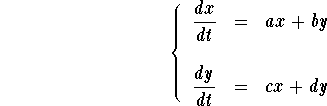In order to find the eigenvalues, consider the characteristic polynomial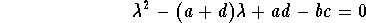.

In this section we will consider the case of the quadratic equation above when it has two distinct real roots (that is, if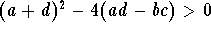). The roots (eigenvalues) are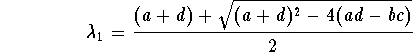,

and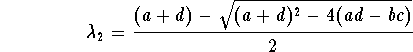.

Here we know that the differential system has two linearly independent straight-line solutions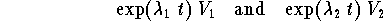,

where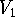(respectively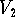) is an eigenvector associated to the eigenvalue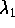(respectively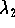). We also know that the general solution (which describes all of the solutions) to the system has the form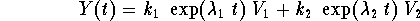.

Keep in mind thatandare two constant vectors.

Let us discuss the behavior of the solutions when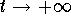(meaning the future) and when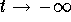(meaning the past). Since the eigenvalues are distinct, one is bigger than the other one. Assume that we have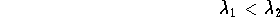.

It is easy to see that we have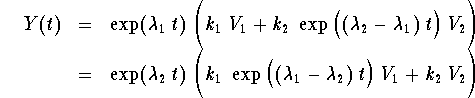Behavior whenIn this case we will consider the equation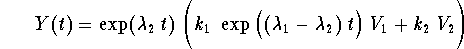.

Since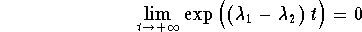,

(because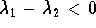) then it is clear that when, we have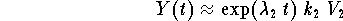.

Behavior whenIn this case we will consider the equation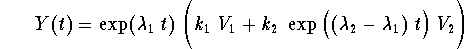Since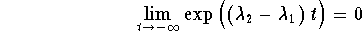(because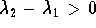) then it is clear that when, we have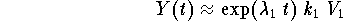Remark:Since the two eigenvalues are real numbers, we have three cases to consider depending on their signs:

Case 1: Both are positive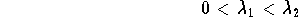.

In this case we have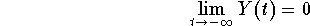,

meaning that the solutions emanate from the origin (if you go to the past, you will die at the origin). When, Y(t) explodes.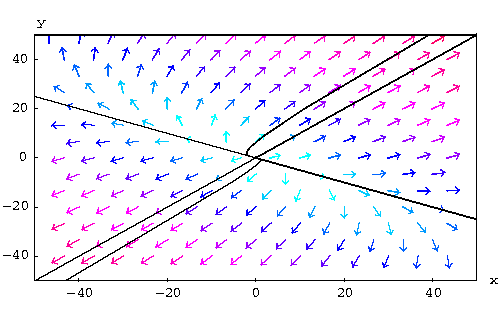In this case the origin plays the role of a source. Clearly, the origin is the only equilibrium point.

Case 2: Both eigenvalues are negative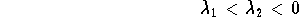.

In this case we have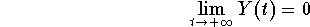,

meaning that in the future the solutions die at the origin. When, Y(t) explodes.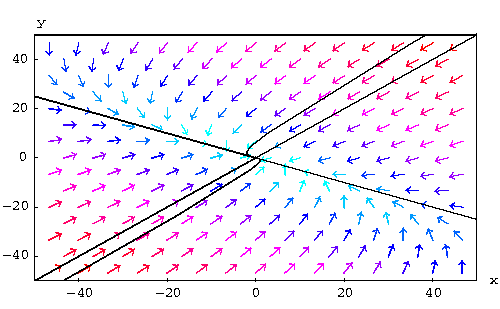In this case, the origin plays the role of a sink. Clearly, the origin is the only equilibrium point.

Case 3: The eigenvalues have different signs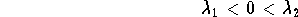.

In this case, the origin behaves like a saddle.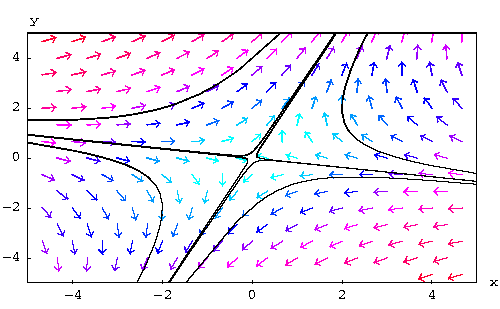Remark: It is clear from the above discussions that one may decide about the signs of the eigenvalues just by looking at some solutions on the phase plane (depending whether we have a saddle, a sink or a source).

Example: Consider the three phase planes and decide about the sign-distribution of the associated eigenvalues.

Phase Plane I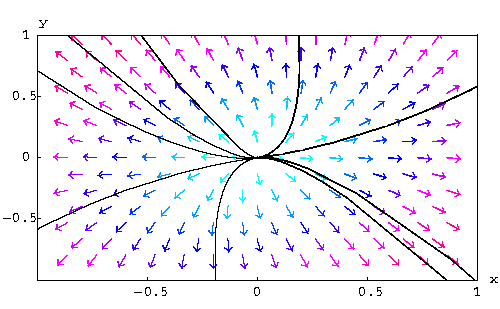Phase Plane II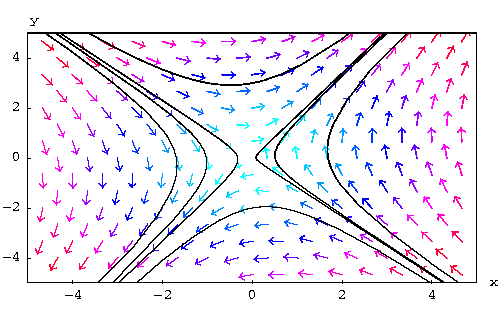Phase Plane III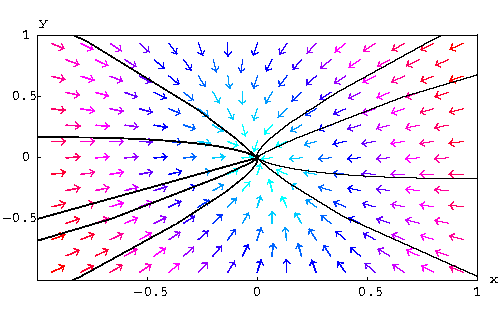For the phase-plane I, the origin is a source. Therefore, the two eigenvalues are both positive.
For the phase-plane II, the origin is a saddle. Hence, the two eigenvalues are opposite signs.
For the phase-plane III, the origin is a sink. Hence, the two eigenvalues are negative.

Example: Consider the harmonic oscillator equation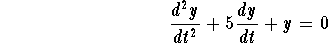.

Discuss the behavior of the spring-mass.

Answer: First, translate this equation to the system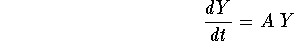,

where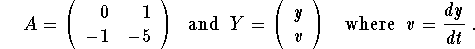The characteristic polynomial of this system is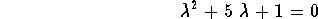.

The eigenvalues are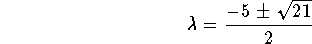.

It is clear that both of them are negative. Hence, the origin is a sink. Meaning that, regardless of the initial condition, the mass will always tend to its equilibrium, or rest, position.
Note that if V is an eigenvector associated to the biggest eigenvalue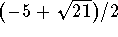, then all the solutions tend to the origin tangent to that vector V. In this case we have.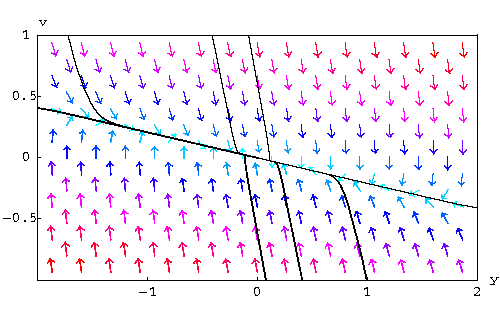Remark: The case when one of the two eigenvalues is zero will be discussed in another section separately.

### If you would like more practice, click on Example.[Differential Equations] [First Order D.E.]
[Geometry] [Algebra] [Trigonometry ]
[Calculus] [Complex Variables] [Matrix Algebra]S.O.S MATHematics home page

Do you need more help? Please post your question on our S.O.S. Mathematics CyberBoard.Author: Mohamed Amine Khamsi